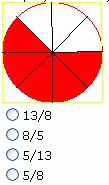Email us to get an instant 20% discount on highly effective K-12 Math & English kwizNET Programs!

#### Online Quiz (WorksheetABCD)

Questions Per Quiz = 2 4 6 8 10

### Grade 2 - Mathematics1.44 Fractions - Without Simplification (WIZ Math)

 Method: Fraction is a way of expressing a quantity based on an amount that is divided into a number of equal-sized parts. Each part is equal to a fraction which is 1/total number of parts. The number on top is called the numerator and the one at the bottom is called the denominator. Example :What is the fraction shown by shaded portion? (Do not simplify fraction) Answer : 5/8 Directions: Answer the following questions. Also write atleast 5 examples of your own.
 Q 1: What is the fraction shown by shaded portion? (Do not simplify fraction)0/11/11/0 Q 2: What is the fraction shown by shaded portion? (Do not simplify fraction)11/83/83/118/3 Q 3: What is the fraction shown by shaded portion? (Do not simplify fraction)4/66/410/64/10 Question 4: This question is available to subscribers only! Question 5: This question is available to subscribers only! Question 6: This question is available to subscribers only! Question 7: This question is available to subscribers only! Question 8: This question is available to subscribers only!